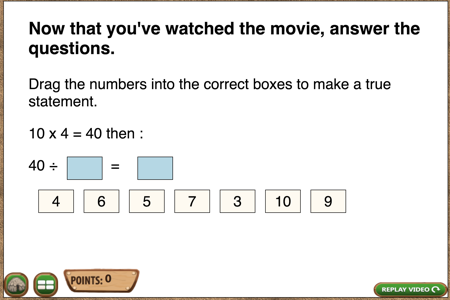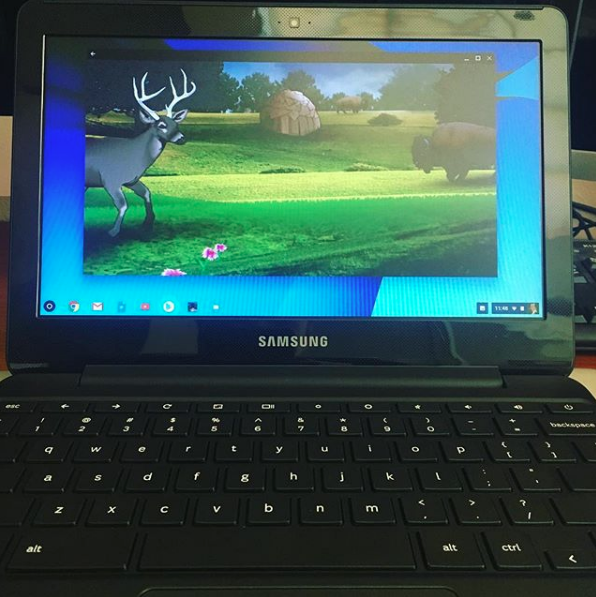# A simple little math problem isn’t so simple

Today, I want to revisit the same math concept, that division is multiplication in reverse, but from a programming standpoint.

First, the setup. The first part sets the number of points from this page to 0 and then reads in the number of points the player already has from playing previously.

We also set the number of points for problem 1 to 0.
``` // RECORDING POINTS SECTION ; let thesepts = 0; if (typeof (sessionStorage.getItem("points")) == 'undefined') { sessionStorage.setItem("points", 0); } let pts = sessionStorage.getItem("points"); let thisone = 0; // The number of points given for this answer ;```

Now we start problem 1, which is this problem:We initialize two variables. One will be the number of boxes we’ve moved, which starts at 0, and the other is the box we are moving right now. Initially, that has no value because we aren’t moving anything.

`// FOR QUESTION 1 ;`

let boxmove = 0;
let thisel; // Which element we’re moving ;

This whole long next thing creates the problem students will answer, including creating the little boxes to drag.

This function does a whole  bunch of things. First, understand that we want 7 boxes of numbers to drag. Two of those should be the correct numbers, but only two, and there should not be the possibility of having two right answers. For example, if the correct answer is 4 x10 =40 , the numbers 5 and 8 should not be among the choices. Also, if the player gets the first answer wrong they shouldn’t be able to continue.

1. It creates an array of the numbers we’ve used, which at first is empty.
2. It sets the number of the correct answers of the numbers picked for the answer boxes (the little boxes to drag) to zero.
3. It selects two random numbers, finds the product of those and then adds the division equation to the page.
4. It randomly selects numbers to be in the choice boxes for dragging. For each number it checks if it is one of the correct answers. If so, it gives this box the class of ‘right’.
5. If we get to the last two boxes and we still haven’t picked the correct number, put the correct numbers in the last two boxes.
6. If we are at the last box and one of the correct numbers hasn’t been picked, put the correct number in the last box.
7. If the correct answer is the same number twice, e.g., 10 x 10, make sure the correct number is in 2 boxes.
8. If there is another possible correct answer, say we want 4 x 10 but 5 x 8 would also work, make sure that no more than one of the numbers from the alternate answer is picked, for example, we can have a 5 or an 8 but not both.
9. Make the little boxes draggable and if a box has a class of ‘right’, give it a value of 1, otherwise give it a value of 0.

At the end of the function, we call it.

### Scoring the answers right or wrong

The next part of this program is to score the answer right or wrong. This happens when it gets dropped into one of the empty boxes. We want to make it evident that they got it right or wrong, so if it is right a ding! sound plays and the box turns green. If wrong, a static sound plays, the box turns red and then the whole problem disappears and the next one appears.

If there are two right answers, the problem slides up and a new problem fades in.

Now mind you, all of this code is for ONE problem to make it more interactive and to minimize confusion for students at the same time. Does one problem make a difference? Probably not, but as I said in the previous post, adding up a little more interactivity 100 times does make a difference in how likely kids are to continue playing.

This problem is in Making Camp Premium – available this summer.  You can get Making Camp free right now, playable in any browser or download from the app store or Google Play.```function createProb1(){
// Initializes array and number of correct answers pushed to the array ;
// Initializes counter to identify other correct answer we don't want ;
let usednums = [];
let anspicked = 0 ;
let suspnum = 0 ;

// Selects two random numbers. Puts those in the problem and the answer boxes ;
// Creates the product of the random numbers and puts those in also ;
let qnum11 =1 +Math.floor((Math.random() * 10)) ;
let qnum12 =1 +Math.floor((Math.random() * 10)) ;
let qnum13 = qnum11 * qnum12 ;
\$("#num1").text(qnum11);
\$("#qnum1").text(qnum11);
\$("#num2").text(qnum12);
\$("#qnum2").text(qnum12);
\$(".num3").text(qnum13);

for (let x=1; x < 8; ) {
// Select random number 1-10, if it is not already used put in the array of used numbers ;
let randx =1 +Math.floor((Math.random() * 10)) ;
if (usednums.indexOf(randx) === -1){
usednums.push(randx);

// If this is one of the correct answers, put it in a box with the class "right" and append to the answers section ;
// Increment number of answers picked and x ;
if (randx === qnum11 || randx === qnum12 ) {
\$("#answers1").append('<div class="smallbox ans draggable right" id="box"'
+ x +' >'+ randx+ '</div>');
x++ ;
anspicked++ ;
}

// Making sure the correct answers are in there  ;
else {
if(anspicked === 0 && x > 5) {
\$("#answers1").append('<div class="smallbox ans draggable right" id="box7">'
+ qnum11+ '</div>');
\$("#answers1").append('<div class="smallbox ans draggable right" id="box8">'
+ qnum12+ '</div>');
anspicked = anspicked + 2 ;
x = 8 ;

}
else  if(anspicked === 1 && x > 6) {
// If it is the same number twice, like 4 x4 , make sure it is there twice ;
if (qnum11 === qnum12){
\$("#answers1").append('<div class="smallbox ans draggable right" id="box8">'+ qnum12+ '</div>');
}
// If it is the first number that hasn't been picked, put that in the last box. Else, put the second number there ;
else if (usednums.indexOf(qnum11) === -1){
\$("#answers1").append('<div class="smallbox ans draggable right" id="box7">'+ qnum11+ '</div>');
}
else if (usednums.indexOf(qnum12) === -1){
\$("#answers1").append('<div class="smallbox ans draggable right" id="box7">'+ qnum12+ '</div>');
}

x = 8 ;

}

// This prevents them from getting two possible right answers, for example, 24 / 6 = 4 and 24/8 = 3 ;
// Only one of those answers is the multiplication problem shown in reverse, though ;
if(qnum13 % randx === 0 ){
if (suspnum === 0) {suspnum = 1 ;}
else {continue;}
}

\$("#answers1").append('<div class="smallbox ans draggable wrong" id="box"' + x +' >' + randx+ '</div>');
x++ ;

}
}```
```\$('.draggable').draggable({
start: function (event, ui) {
if (\$(this).hasClass('right')) {
thisone = 1;
thisel = this;
}
else {
thisone = 0;
thisel = this;
wrongel = this }
}
});
}
}

// EXECUTE THE FUNCTION THAT CREATES THE PROBLEM ;```
```createProb1() ;

\$('.ansq1').droppable({
drop: function (event, ui) {
boxmove++;
pts = eval(pts) + thisone;
thesepts += thisone;

\$("#numberpts").text(pts);

\$(thisel).draggable('disable');
if (thisone == 1) {
pointy();
\$(thisel).css({ 'background-color': 'green' });
playAudio('ding_right');
}
else {
\$(thisel).css({ 'background-color': 'red' });
playAudio('static_wrong');
setTimeout(function(){
\$('#q1').hide();
{ \$("#q2").slideDown('slow'); }
}, 1200);
}
if (boxmove === 2) {
setTimeout(function(){
\$('#q1').hide();
{ \$("#q2").slideDown('slow'); }
}, 1200);
}
}
});```